# SAT II Math II : Data Analysis and Statistics

## Example Questions

1 2 4 Next →

### Example Question #2 : Probability

What is the probability of choosing 2 67's in a row out of the following data set? Assume replacement.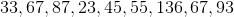Possible Answers: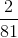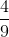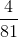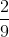Correct answer:Explanation:

What is the probability of choosing 2 67's in a row out of the following data set? Assume replacement.Let's begin by recalling that probability can be thought of as follows: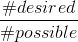Meaning that the probability of an event is the number of times the desired outcome exists, over the total number of outcomes.

In this case, each pick has two possible desired outcomes, and 9 total outcomes. (there are two 67's, and 9 total terms).

So the probability of each choice being a 67 is:However, we are not done yet. We need the probability of choosing two 67's in a row. Assuming replacement means that each probability will be the same, so to find the probability of events, we will multiply each probability together. This gets: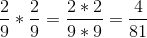### Example Question #3 : Probability

A fair coin is tossed 4 times. What is the total number ways the results can be observed (ie HHHH, HHHT, HHTH.....).

Possible Answers:Correct answer:Explanation:

In general, the number of outcomes that can be observed when flipping a coin is found using the formula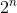where n is the number of tosses. In this case there are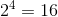ways to observe the outcome.

Alternatively, if n is small enough you can list out the possible outcomes:

1. HHHH

2. HHHT

3. HHTH

4. HTHH

5. THHH

6. HHTT

7. HTHT

8. HTTH

9. THTH

10. TTHH

11. THHT

12. TTTH

13. TTHT

14. THTT

15. HTTT

16. TTTT

### Example Question #2 : Probability

In a carnival game, a player must spin two independent spinners. If the first spinner has a probability oflanding on red, and the second spinner has a probability of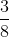landing on red, what is the probability of the first spinner NOT landing on red, and the second spinner landing on red?

Possible Answers: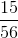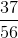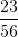Correct answer:Explanation:

Since the spinners are independent of each other, we will need to multiply the probability of not landing on red on the first spinner and the probability of landing on red on the second spinner together.

The probability of not landing on red for the first spinner is found by subtracting the probability of landing on red from.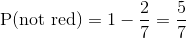Thus,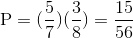### Example Question #5 : Probability

Suppose there is a bag of marbles.  There are two reds, two greens, and one orange marbles.  Without replacement, what is the probability of choosing a red, and another red?

Possible Answers: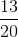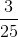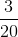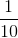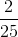Correct answer:Explanation:

There are five marbles altogether.

The first marble is a red, which is two of the five marbles in the bag.

The probability of choosing a red for the first trial is:Without replacement, there are four marbles remaining, and only one red marble remains.

The probability of choosing a red for the second trial is:Multiply the two probabilities.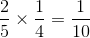The answer is:### Example Question #1 : Representing Data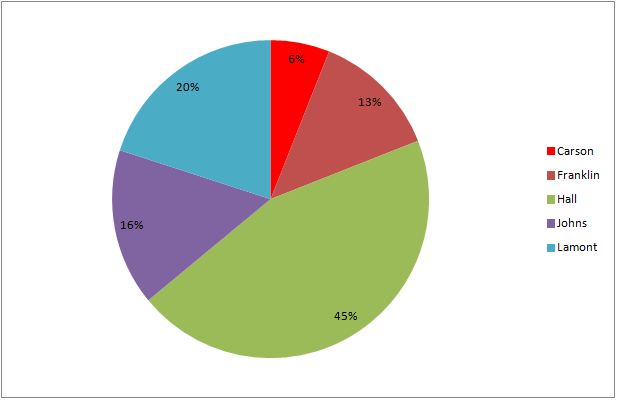The above graph represents the results of a general election for mayor of Kingston.

If 1,658 people voted for Carson, then which comes closest to the number of people who voted for Franklin?

Possible Answers: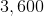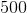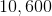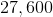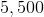Correct answer:Explanation:

The ratio of Franklin's percent of the vote to Carson's is the same as the number of votes for Franklin to those for Carson. From the information in the graph, settingto the number of votes for Franklin,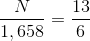Multiply both sides by 1,658 and solve: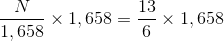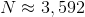The closest estimate would be 3,600 votes.

### Example Question #2 : Representing Data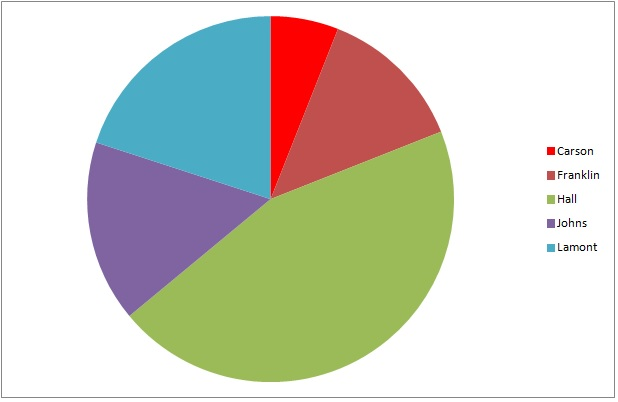The above graph represents the results of a general election for mayor of Kingston.

If 8,349 people voted, which of the following numbers is closest to the number of voters who did not vote for the winning candidate?

Possible Answers: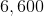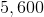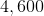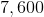Correct answer:Explanation:

The four wedges representing the four nonwinners - all except the largest wedge, which is green - together make up slightly more than half the circle. 55% is a good estimate, and 55% of 8,349 is equal to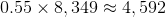The best estimate of the given choices is 4,600.

### Example Question #1 : Other Data Analyses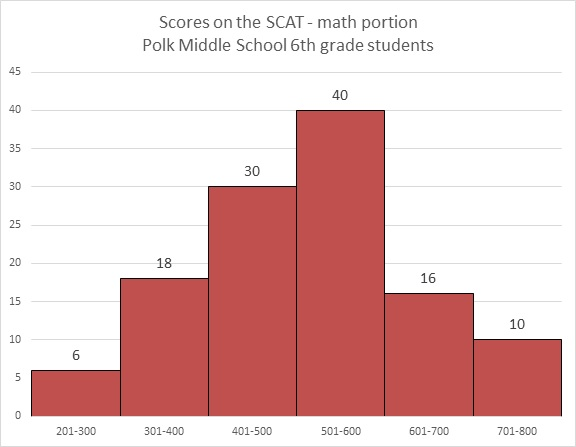Refer to the above diagram. Maureen achieved a score of 603, which was the lowest score in the 601-700 range. Which of the following comes closest to being her percentile score?

Possible Answers: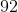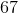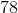Correct answer:Explanation:

The histogram represents a total of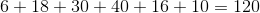scores.

Maureen's percentile is the percent of the scores that she outscored. Those scores were exactly the ones in the first four intervals shown - that is,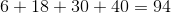out of the 120 scores, which was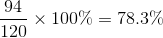of the scores.

Percentile is given in whole numbers, so round this to 78.

### Example Question #2 : Other Data Analyses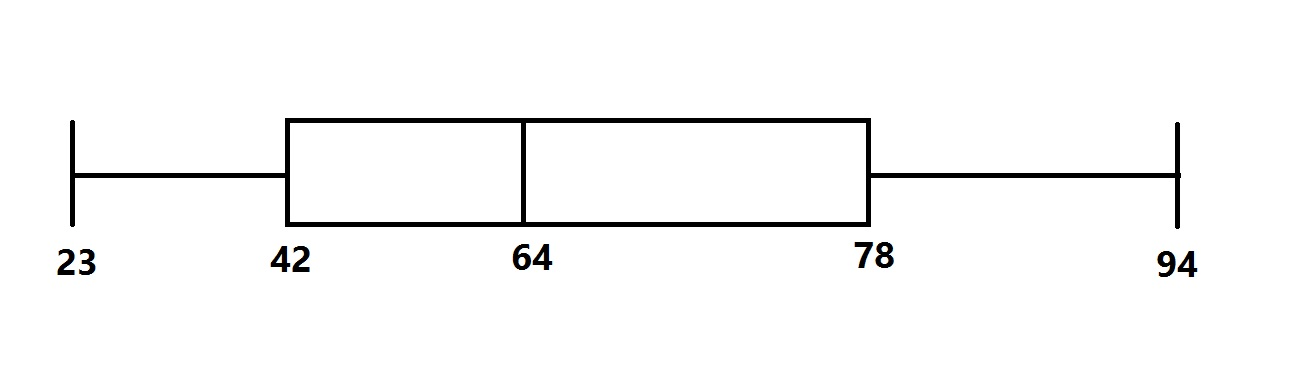The distribution of scores for a test given to a large group of students is represented by the above box-and-whisker plot.

Which of the following questions cannot be answered from the diagram without looking for other information?

Possible Answers:

What is the range of the test scores?

What is the mean of the test scores?

What is the median of the test scores?

What is the interquartile range of the test scores?

What is the midrange of the test scores?

Correct answer:

What is the mean of the test scores?

Explanation:

The midrange of the scores is the mean of the least and greatest scores, which are represented by the "whiskers" at either end: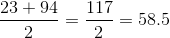The range of the scores is the difference of the least and greatest scores: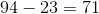The median of the scores is represented by the vertical line within the box -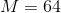The interquartile range is the difference of the third and first quartiles, which are represented by the ends of the box: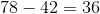Note that these scores all depend on the positions of the scores. The mean, however, depends on the scores themselves, which are not reflected in the diagram. The question cannot be answered from the box-and-whisker plot.

### Example Question #2 : Other Data AnalysesRefer to the above diagram. Which of the following scores could possibly be at the eightieth percentile?

Possible Answers: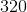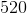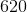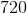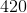Correct answer:Explanation:

The histogram represents a total ofscores.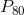, the 80th percentile, is the score that ranks above 80% of the scores, which, here, is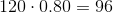scores.

This score would outrank thescores in the first four ranges graphed, but not all of the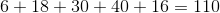scores in the first five ranges.

This score must fall in the fifth range shown, which is the 601-700 range.

Therefore, the correct choice of the ones given is 620.

1 2 4 Next →

### All SAT II Math II Resources